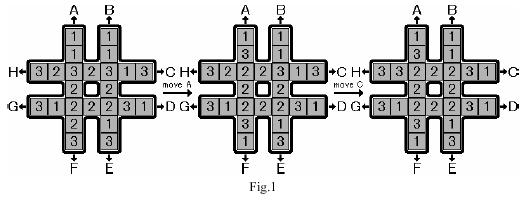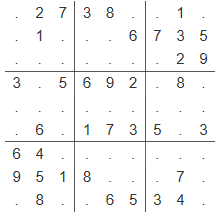# 搜索

# Acwing181 回转游戏……

……

……

# Acwing170 加成序列

1、$X = 1$

2、$X[m] = n$

3、$X < X < … < X[m-1] < X[m]$

4、对于每个 $k(2≤k≤m)$都存在两个整数 $i$ 和 $j$ （$1≤i,j≤k−1$，$i$ 和 $j$ 可相等），使得 $X[k] = X[i] + X[j]$。

……

……

# Acwing166 数独……